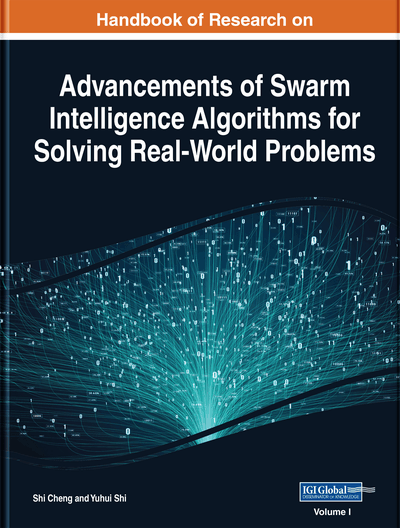# Evaluation of Bayesian Network Structure Learning Using Elephant Swarm Water Search Algorithm

Shahab Wahhab Kareem (Erbil Polytechnic University, Iraq) and Mehmet Cudi Okur (Yaşar University, Turkey)
DOI: 10.4018/978-1-7998-3222-5.ch008

## Abstract

Bayesian networks are useful analytical models for designing the structure of knowledge in machine learning which can represent probabilistic dependency relationships among the variables. The authors present the Elephant Swarm Water Search Algorithm (ESWSA) for Bayesian network structure learning. In the algorithm; Deleting, Reversing, Inserting, and Moving are used to make the ESWSA for reaching the optimal structure solution. Mainly, water search strategy of elephants during drought periods is used in the ESWSA algorithm. The proposed method is compared with Pigeon Inspired Optimization, Simulated Annealing, Greedy Search, Hybrid Bee with Simulated Annealing, and Hybrid Bee with Greedy Search using BDeu score function as a metric for all algorithms. They investigated the confusion matrix performances of these techniques utilizing various benchmark data sets. As presented by the results of evaluations, the proposed algorithm achieves better performance than the other algorithms and produces better scores as well as the better values.
Chapter Preview
Top

## Introduction

Probabilistic graphical models, have been to be valuable instruments as a description of ambiguous knowledge. The theory of probability presents methods to analyze how the components joined, guaranteeing that the system remains consistent. The combined results expected to be compatible and present new techniques to propose new interface models for observed data. The graph-theoretic side of graphical models presents both an appealing interface within which humans can create interacting collections of variables and a data structure that can use in powerful general-purpose algorithms (Friedman, Murphy, & Russell, 1998). Bayesian networks (Koski & Noble, 2009; Pourret & Naim, 2008) are such a kind of probabilistic graphical models.

Bayesian networks (BN) are one of the simplified analytical methods for constructing the probabilistic structure of knowledge in machine learning (Ji, Wei, Liu, 2012). They can be implemented universally in knowledge design, argumentation, and inference (Fortier, Sheppard & Pillai, 2013). The structure of Bayesian network is a direct acyclic graph (DAG) which formed concerning two significant parts; the parameters and the structure of the network. The parameters describe conditional probabilities, and the structure expresses dependencies among the variables. Solving the learning structure of a Bayesian network without a suitable search method is difficult. The challenges for learning the optimal structure of a Bayesian network (BN) from a dataset is an NP-hard optimization problem (Li & Chen, 2014); however, extensive research conducted to develop approximate strategies for learning network structure. Essentially, there are two procedures for Bayesian networks structural learning. The first is a constraint-based procedure while the second is score and search procedure (Margaritis, 2003). The score and search method is used to explore the space of BN structures and continuously evaluate all applicant network structures until the valid metric value achieved.

Score-based procedures rely on a function to evaluate the network, the available data, and they search for a structure that optimizes the score, which is the goal (Fast, 2010). The score function method implemented using two primary criteria: Bayesian score and information-theoretic score. The information-theoretic score has implemented in methods like; Log-likelihood (LL), Akaike information criterion (AIC), Bayesian Information Criterion (BIC), Minimum Description Length (MDL), Normalized Minimum Likelihood (NML), and Mutual Information Tests (MIT). The Bayesian score has implemented in other methods like; BD (Bayesian Dirichlet), BDeu (Bayesian Dirichlet (“e” for likelihood-equivalence)), BDeu (Bayesian Dirichlet equivalent uniform (“u” for uniform joint distribution)), and K2 (Cooper & Herskovits, 1992).

## Complete Chapter List

Search this Book:
Reset